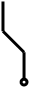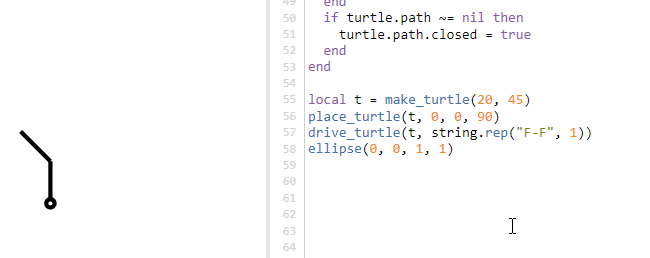# Blog » Drawing simple generative organics with L-systems

Today we’ll take a brief break from technical diagramming to talk about something fun: drawing simple generative tree-like structures with Lua using a concept called an L-system.

### What is an L-system?

An L-system, or Lindenmayer system, is a mechanism used to describe the natural growth behavior of some types of organisms (like plants or trees). With an L-system, you can encode a simple set of rules which, when applied iteratively, result in arbitrarily complex geometric structures, like this:A tree-like structure generated with an L-system.

With an L-system, you specify the starting state of the diagram (usually called the “axiom”) and a set of simple transformation rules that modify the state of the diagram with each application. The result after a few applications of the rule transformations is a simple string of drawing commands that place lines on the screen.

First we’ll look at some simpler examples to learn how L-systems work, and then we’ll play around with them in Vexlio using the interactive Lua program mode.

### Turtle motivation

Suppose you had a pen attached to a machine to which you can give simple commands like “move forward 10 units” or “turn left 45°”. By following a list of these commands, the machine will drag the pen across the paper, drawing lines as it moves. Traditionally, this method of producing graphics is referred to as “turtle graphics,” as the machine moving the pen was originally a little mechanical turtle.

Let’s say the character “F” stands for the command to “move forward 10 units.” Let’s also say “-” stands for “turn left 45°”, and “+” stands for “turn right 45°.” Then the string of characters “F-F+F” would produce the following path (assuming the turtle starts at the circle, facing upwards):F-F+F

With just these three simple commands, the turtle can already produce some fairly interesting drawings, and program mode’s live-update as you type feature makes it easy to experiment:F-F+FF++FF-F+FF++FF-F+FF++FF-F+FF++F in Vexlio's Lua program mode

Creating drawings with this simple turtle system is already a fun thing to play around with, and you can grab the turtle .vex file and a free Vexlio trial to do just that.

### Turtle branching

So far so good, but things start to get really interesting when we add the concept of “branches” that the turtle can take. With branches, we allow the turtle to place a bookmark to remember its current position and orientation, execute a sequence of commands, and then jump back to its previous place by looking at the bookmark. We’ll add two new turtle commands, “[” (open bracket) which means “place bookmark” and “]” (close bracket) which means “go back to previous bookmark.”

For example, the turtle directions “F[-F][+F]F” would look like this:F[-F][+F]F branching turtle

At the first “[” command, the turtle remembers its position and orientation. Next, it follows the “-F” commands, turning left and moving forward to create the leftmost branch. Then it encounters the “]” command, so it resumes its previous position and orientation before executing the rest of the commands. Download the branching turtle .vex file.

### L-system productions

So far we’ve just talked about making simple drawings by creating lists of commands for the turtle to follow. The power of L-systems comes from the system of rules you create to generate directions for the turtle to follow.

Every L-system starts with an initial set of turtle directions called the “axiom.” Additionally, every L-system has rules that transform commands in the directions string into other commands. For example, suppose the axiom is “F”, i.e. the turtle will just move forward and that’s it. If we supply the rule “F” → “F-F” and apply the rule several times, the directions are transformed by that rule each step to arrive at a final result:

Step # Directions
0 "F" (the axiom)
1 "F-F"
2 "F-F-F-F"
3 "F-F-F-F-F-F-F-F"

The concept is fairly simple: each step in the process just replaces all occurrences of “F” (in the previous step’s result) with “F-F.” As the rule is applied more than once, the directions string develops according to the replacement rules.

We now have everything we need to create a turtle-driven L-system. The simple L-system with axiom “F” and rule “F” → “F[-F][+F]” and a turtle turn angle of 25°, we can produce the following simple tree after 5 applications of the rule:F → F[-F][+F]

One final clever trick we can use is to introduce new “dummy” commands into the rule set, which we can use to control how the directions string evolves. While the end result for the directions string may contain leftover dummy commands, we’ll have the turtle just ignore them when it encounters them. With dummy commands giving us more options for evolving the directions, we can start to generate more organic-appearing structures:Axiom X; F → FF and X → -F[+F][---X]+F-F[++++X]-X. Note "X" is a dummy command.

Now that we have the basic setup, we can play around with rule sets to generate more interesting structures. Here are some other examples:Axiom X; F → FF and X → F+[-F-XF-X][+FF][--XF[+X]][++F-X]Axiom X; F → FX[FX[+XF]] and X → FF[+XZ++X-F[+ZX]][-X++F-X] and Z → [+F-X-F][++ZX]Axiom X; F → FXF[-F[-FX]+FX] and X → F++F

### Other structures

L-systems, while originally used to describe biological structures, can also be used to construct other recursively-defined structures. For example, with the same turtle-based system we used for generating tree-like structures, we can also generate the following repeating pattern of rectangles (from “Graphical modeling using L-systems,” by Algorithmic Botany):Axiom F-F-F-F; F → FF-F+F-F-FF

The Sierpinksi Triangle can be drawn if we define another command “G” that is synonymous with “F”:Axiom F-G-G; F → F-G+F+G-F and G → GG

Although the “G” command is interpreted by the turtle the same as “F”, introducing it into the rule set gives us more options to control how different parts of the command string evolve over time (similar to the earlier use of dummy commands).

### Drawing L-Systems with Lua

A turtle-based Lua drawing system for L-systems is quite easy in Vexlio’s program mode.

To follow along, create a new drawing and convert it to program mode using the “File → Convert Drawing to Program Mode” menu item. This will give us a blank canvas and a Lua editor to start writing code. (For a separate tutorial on program mode, you can see our User Guide. You can learn more about the Lua drawing API on the API reference page).

We’ll start by defining a simple function to create a turtle “object” (which is just a Lua table):

 ```1 2 3 4 5 6``` ```-- Create a new turtle "object" with the given parameters. -- step_size: How far the turtle moves with the F command. -- angle_inc: The turn amount (in degrees) for +/- commands. function make_turtle(step_size, angle_inc) return {d=step_size, a=math.rad(angle_inc), width=2} end ```

Then we’ll create a mapping of our turtle commands (“F”, “+”, “-”, etc) to functions that can be used to execute each of them:

 ``` 7 8 9 10 11 12``` ```-- Mapping of command character to turtle action. local actions = { ["F"] = function(turtle) draw_forward(turtle) end, ["-"] = function(turtle) left(turtle) end, ["+"] = function(turtle) right(turtle) end } ```

Then, “driving” a turtle is as simple as looking at each character in its directions string, and executing the appropriate function from the `actions` table:

 ```13 14 15 16 17 18 19 20 21``` ```-- Drive the turtle according to the given direction string. function drive_turtle(turtle, directions) for char in directions:gmatch(".") do local fnc = actions[char] if fnc ~= nil then fnc(turtle) end end end ```

Finally, we’ll define the functions that actually perform the turtle actions of “draw forward,” “turn left,” and “turn right”:

 ```22 23 24 25 26 27 28 29 30 31 32 33 34 35 36 37 38 39 40``` ```-- Draw a path from the turtles current position to its next position, -- taking into account its step size and current direction. function draw_forward(turtle) local new_x = turtle.x + turtle.d * math.cos(turtle.facing) local new_y = turtle.y - turtle.d * math.sin(turtle.facing) local p = path(turtle.x, turtle.y, new_x, new_y) p.strokeWidth = turtle.width turtle.x, turtle.y = new_x, new_y end -- Turn the turtle right (CCW) by its angle increment. function right(turtle) turtle.facing = turtle.facing - turtle.a end -- Turn the turtle left (CW) by its angle increment. function left(turtle) turtle.facing = turtle.facing + turtle.a end ```

All that’s left to do now is create a turtle object, place it in some starting position, and give a directions string to the `drive_turtle` function:

 ```41 42 43 44 45``` ```local directions = "FF-F+F-F-FF" local t = make_turtle(20, 90) t.x, t.y, t.facing = 0, 0, 0 drive_turtle(t, directions) ellipse(0, 0, 1, 1) -- Mark starting position ```Turtle drawing from the above Lua code.

This is enough for a simple turtle-based drawing system. To fully implement L-system drawing, we need one additional function which, given a set of rules, applies them to a directions string and returns the result:

 ```41 42 43 44 45 46 47 48 49 50 51 52 53 54 55 56 57 58 59 60``` ```-- Run the L-system productions n times on the given "initial" string (the axiom). -- Return the resulting command string. function run_productions(initial, productions, n) local acc = initial for i = 1, n do -- For each char in acc, replace it and append to new acc. -- then swap acc = newacc local newacc = "" for char in acc:gmatch(".") do local repl = productions[char] if repl ~= nil then newacc = newacc .. repl else newacc = newacc .. char end end acc = newacc end return acc end ```

We now have an L-system drawing mechanism. For example, to produce the repeating rectangles L-system from an earlier example, all we have to do is encode the rules and run the productions:

 ```61 62 63 64 65 66 67``` ```local directions = run_productions("F-F-F-F", { ["F"] = "FF-F+F-F-FF" }, 3) local t = make_turtle(20, 90) t.x, t.y, t.facing = 0, 0, 0 drive_turtle(t, directions) ellipse(0, 0, 1, 1) -- Mark starting position ```Repeating rectangles L-system.

The only thing left to do is to add the branching capabilities. All we need to do is add support for the turtle branching commands using a simple stack mechanism, and add them to the `actions` table:

 ``` 7 8 9 10 11 12 13 14 15 16 17 18 19 20 21 22 23 24 25 26 27 28 29 30 31 32 33``` ```-- Mapping of command character to turtle action. -- Push the current turtle state to the top of a stack. function push(turtle) if turtle.stack == nil then turtle.stack = {} end table.insert(turtle.stack, {x = turtle.x, y = turtle.y, facing = turtle.facing, width = turtle.width}) end -- Pop the turtle state from the tock of the stack and apply it. function pop(turtle) local val = turtle.stack[#turtle.stack] table.remove(turtle.stack) turtle.x = val.x turtle.y = val.y turtle.facing = val.facing turtle.width = val.width end local actions = { ["F"] = function(turtle) draw_forward(turtle) end, ["-"] = function(turtle) left(turtle) end, ["+"] = function(turtle) right(turtle) end, ["["] = function(turtle) push(turtle) end, ["]"] = function(turtle) pop(turtle) end } ```

We can now use the branching commands in our production rules, such as in the example from earlier:F → F[-F][+F]